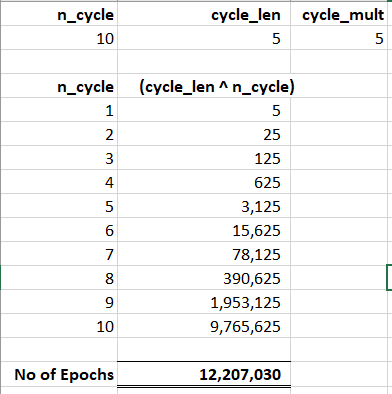# Understanding cycle_len and cycle_mult

In the code learn.fit(lr, 3, cycle_len=1, cycle_mult=2), can you please explain the function of cycle_len and cycle_mult?

Also, is 3 the number of epochs?

1 Like

@himani, these notes might help to explain the difference

3 Likes

The `cycle_len` and `cycle_mult` parameters are used for doing a variation on stochastic gradient descent called “stochastic gradient descent with restarts” (SGDR).

This blog post by @mark-hoffmann gives a nice overview, but briefly, the idea is to start doing our usual minibatch gradient descent with a given learning rate (`lr`), while gradually decreasing it (the fast.ai library uses “cosine annealing”)… until we jump it back up to `lr`!

The `cycle_len` parameter governs how long we’re going to ride that cosine curve as we decrease… decrease… decrease… the learning rate. Cycles are measured in epochs, so `cycle_len=1` by itself would mean to continually decrease the learning rate over the course of one epoch, and then jump it back up. The `cycle_mult` parameter says to multiply the length of a cycle by something (in this case, 2) as soon as you finish one.

So, here we’re going to do three cycles, of lengths (in epochs): 1, 2, and 4. So, 7 epochs in total, but our SGDR only restarts twice.

36 Likes

Thank you so much, it is very helpfulThank you very much for sharing the notesPrefer giving a search in the forum…
Thanks…

Whilst that’s true, it’s important to note that some conceptual ideas are hard to search for and digest.

3 Likes

That’s true…
But I too learnt this search first and then ask concept quite helpful…
Learnt from this amazing forum itself…

Here’s the reference link…(Scrolling a bit below the answers my doubt too)Image credit @Moody

3 Likes

some conceptual ideas are hard to search for and digest

Totally agree. Speaking as someone who audited p1v1 and took p1v2 live, it was so much easier to make use of the forums while the course was live. Part of it was being involved, but also, searching for keywords over the entire course becomes tricky.

Video timings and wiki-ified stuff make it a lot easier

I searched the forums, however could not quite understand the answers to a related (but different) question.

Super helpful. I wonder whether cycle_len be better named “`num_epochs_per_cycle`”?

3 Likes

which epoch are we talking about here
Models training epoch ?
if yes then m not sure at what point of time does keras calls for method for decaying the LR in a cosine cycle
if it have to interpret epoch as training epoch then what it could mean is we are going to start with lesser max value of LR in next cycle…

def on_epoch_end(self, epoch, logs={}):
‘’‘Check for end of current cycle, apply restarts when necessary.’’’
if epoch + 1 == self.next_restart:
self.batch_since_restart = 0
self.cycle_length = np.ceil(self.cycle_length * self.mult_factor)
self.next_restart += self.cycle_length
self.max_lr *= self.lr_decay
self.best_weights = self.model.get_weights()